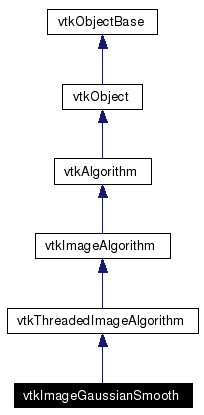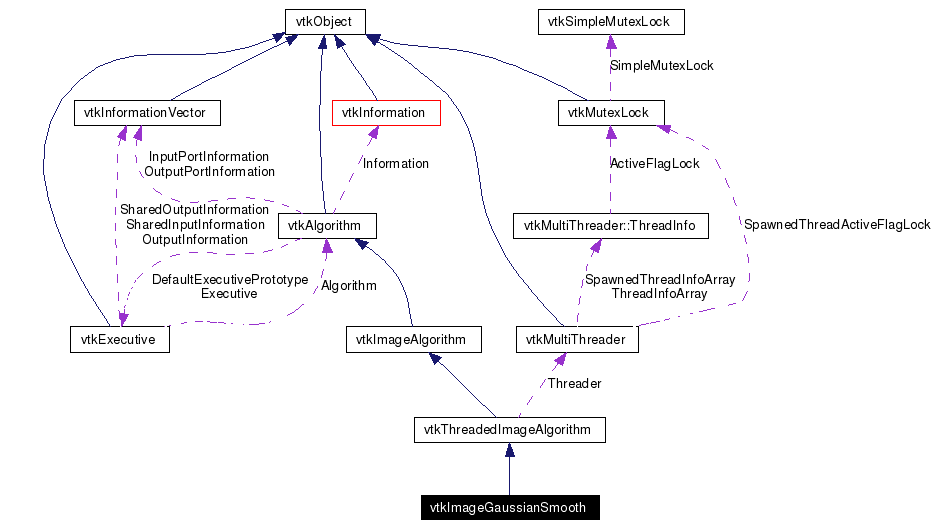# vtkImageGaussianSmooth Class Reference

`#include <vtkImageGaussianSmooth.h>`

Inheritance diagram for vtkImageGaussianSmooth:[legend]
Collaboration diagram for vtkImageGaussianSmooth:[legend]
List of all members.

## Detailed Description

Performs a gaussian convolution.

vtkImageGaussianSmooth implements a convolution of the input image with a gaussian. Supports from one to three dimensional convolutions.

Tests:
vtkImageGaussianSmooth (Tests)

Definition at line 31 of file vtkImageGaussianSmooth.h.
virtual void SetStandardDeviations (double, double, double)
virtual void SetStandardDeviations (double)
void SetStandardDeviation (double std)
void SetStandardDeviations (double a, double b)
virtual double * GetStandardDeviations ()
virtual void GetStandardDeviations (double &, double &, double &)
virtual void GetStandardDeviations (double)
static vtkImageGaussianSmoothNew ()

## Public Member Functions

virtual const char * GetClassName ()
virtual int IsA (const char *type)
void PrintSelf (ostream &os, vtkIndent indent)
void SetStandardDeviation (double a, double b)
void SetStandardDeviation (double a, double b, double c)
virtual void SetRadiusFactors (double, double, double)
void SetRadiusFactors (double f, double f2)
virtual void GetRadiusFactors (double &, double &, double &)
virtual void SetDimensionality (int)
virtual int GetDimensionality ()

## Static Public Member Functions

static int IsTypeOf (const char *type)
static vtkImageGaussianSmoothSafeDownCast (vtkObject *o)

## Protected Member Functions

vtkImageGaussianSmooth ()
~vtkImageGaussianSmooth ()
void ComputeKernel (double *kernel, int min, int max, double std)
virtual int RequestUpdateExtent (vtkInformation *, vtkInformationVector **, vtkInformationVector *)
void InternalRequestUpdateExtent (int *, int *)
void ExecuteAxis (int axis, vtkImageData *inData, int inExt, vtkImageData *outData, int outExt, int *pcycle, int target, int *pcount, int total, vtkInformation *inInfo)
void ThreadedRequestData (vtkInformation *request, vtkInformationVector **inputVector, vtkInformationVector *outputVector, vtkImageData ***inData, vtkImageData **outData, int outExt, int id)

## Protected Attributes

int Dimensionality
double StandardDeviations 

## Member Typedef Documentation

 Reimplemented from vtkThreadedImageAlgorithm. Definition at line 34 of file vtkImageGaussianSmooth.h.

## Constructor & Destructor Documentation

 vtkImageGaussianSmooth::vtkImageGaussianSmooth ( ) ` [protected]`

 vtkImageGaussianSmooth::~vtkImageGaussianSmooth ( ) ` [protected]`

## Member Function Documentation

 virtual const char* vtkImageGaussianSmooth::GetClassName ( ) ` [virtual]`

 static int vtkImageGaussianSmooth::IsTypeOf ( const char * type ) ` [static]`
 Return 1 if this class type is the same type of (or a subclass of) the named class. Returns 0 otherwise. This method works in combination with vtkTypeRevisionMacro found in vtkSetGet.h. Reimplemented from vtkThreadedImageAlgorithm.

 virtual int vtkImageGaussianSmooth::IsA ( const char * type ) ` [virtual]`
 Return 1 if this class is the same type of (or a subclass of) the named class. Returns 0 otherwise. This method works in combination with vtkTypeRevisionMacro found in vtkSetGet.h. Reimplemented from vtkThreadedImageAlgorithm.

 static vtkImageGaussianSmooth* vtkImageGaussianSmooth::SafeDownCast ( vtkObject * o ) ` [static]`

 void vtkImageGaussianSmooth::PrintSelf ( ostream & os, vtkIndent indent ) ` [virtual]`
 Methods invoked by print to print information about the object including superclasses. Typically not called by the user (use Print() instead) but used in the hierarchical print process to combine the output of several classes. Reimplemented from vtkThreadedImageAlgorithm.

 static vtkImageGaussianSmooth* vtkImageGaussianSmooth::New ( ) ` [static]`
 Creates an instance of vtkImageGaussianSmooth with the following defaults: Dimensionality 3, StandardDeviations( 2, 2, 2), Radius Factors ( 1.5, 1.5, 1.5) Reimplemented from vtkAlgorithm.

 virtual void vtkImageGaussianSmooth::SetStandardDeviations ( double , double , double ) ` [virtual]`
 Sets/Gets the Standard deviation of the gaussian in pixel units.

 virtual void vtkImageGaussianSmooth::SetStandardDeviations ( double  ) ` [virtual]`
 Sets/Gets the Standard deviation of the gaussian in pixel units.

 void vtkImageGaussianSmooth::SetStandardDeviation ( double std ) ` [inline]`
 Sets/Gets the Standard deviation of the gaussian in pixel units. Definition at line 46 of file vtkImageGaussianSmooth.h.

 void vtkImageGaussianSmooth::SetStandardDeviations ( double a, double b ) ` [inline]`
 Sets/Gets the Standard deviation of the gaussian in pixel units. Definition at line 48 of file vtkImageGaussianSmooth.h.

 virtual double* vtkImageGaussianSmooth::GetStandardDeviations ( ) ` [virtual]`
 Sets/Gets the Standard deviation of the gaussian in pixel units.

 virtual void vtkImageGaussianSmooth::GetStandardDeviations ( double & , double & , double & ) ` [virtual]`
 Sets/Gets the Standard deviation of the gaussian in pixel units.

 virtual void vtkImageGaussianSmooth::GetStandardDeviations ( double  ) ` [virtual]`
 Sets/Gets the Standard deviation of the gaussian in pixel units.

 void vtkImageGaussianSmooth::SetStandardDeviation ( double a, double b ) ` [inline]`
 Sets/Gets the Standard deviation of the gaussian in pixel units. These methods are provided for compatibility with old scripts Definition at line 56 of file vtkImageGaussianSmooth.h.

 void vtkImageGaussianSmooth::SetStandardDeviation ( double a, double b, double c ) ` [inline]`
 Sets/Gets the Standard deviation of the gaussian in pixel units. These methods are provided for compatibility with old scripts Definition at line 58 of file vtkImageGaussianSmooth.h.

 virtual void vtkImageGaussianSmooth::SetRadiusFactors ( double , double , double ) ` [virtual]`
 Sets/Gets the Radius Factors of the gaussian (no unit). The radius factors determine how far out the gaussian kernel will go before being clamped to zero.

 virtual void vtkImageGaussianSmooth::SetRadiusFactors ( double  ) ` [virtual]`
 Sets/Gets the Radius Factors of the gaussian (no unit). The radius factors determine how far out the gaussian kernel will go before being clamped to zero.

 void vtkImageGaussianSmooth::SetRadiusFactors ( double f, double f2 ) ` [inline]`
 Sets/Gets the Radius Factors of the gaussian (no unit). The radius factors determine how far out the gaussian kernel will go before being clamped to zero. Definition at line 67 of file vtkImageGaussianSmooth.h.

 void vtkImageGaussianSmooth::SetRadiusFactor ( double f ) ` [inline]`
 Sets/Gets the Radius Factors of the gaussian (no unit). The radius factors determine how far out the gaussian kernel will go before being clamped to zero. Definition at line 69 of file vtkImageGaussianSmooth.h.

 virtual double* vtkImageGaussianSmooth::GetRadiusFactors ( ) ` [virtual]`
 Sets/Gets the Radius Factors of the gaussian (no unit). The radius factors determine how far out the gaussian kernel will go before being clamped to zero.

 virtual void vtkImageGaussianSmooth::GetRadiusFactors ( double & , double & , double & ) ` [virtual]`
 Sets/Gets the Radius Factors of the gaussian (no unit). The radius factors determine how far out the gaussian kernel will go before being clamped to zero.

 virtual void vtkImageGaussianSmooth::GetRadiusFactors ( double  ) ` [virtual]`
 Sets/Gets the Radius Factors of the gaussian (no unit). The radius factors determine how far out the gaussian kernel will go before being clamped to zero.

 virtual void vtkImageGaussianSmooth::SetDimensionality ( int ) ` [virtual]`
 Set/Get the dimensionality of this filter. This determines whether a one, two, or three dimensional gaussian is performed.

 virtual int vtkImageGaussianSmooth::GetDimensionality ( ) ` [virtual]`
 Set/Get the dimensionality of this filter. This determines whether a one, two, or three dimensional gaussian is performed.

 void vtkImageGaussianSmooth::ComputeKernel ( double * kernel, int min, int max, double std ) ` [protected]`

 virtual int vtkImageGaussianSmooth::RequestUpdateExtent ( vtkInformation * , vtkInformationVector ** , vtkInformationVector * ) ` [protected, virtual]`
 Reimplemented from vtkImageAlgorithm.

 void vtkImageGaussianSmooth::InternalRequestUpdateExtent ( int * , int * ) ` [protected]`

 void vtkImageGaussianSmooth::ExecuteAxis ( int axis, vtkImageData * inData, int inExt, vtkImageData * outData, int outExt, int * pcycle, int target, int * pcount, int total, vtkInformation * inInfo ) ` [protected]`

 void vtkImageGaussianSmooth::ThreadedRequestData ( vtkInformation * request, vtkInformationVector ** inputVector, vtkInformationVector * outputVector, vtkImageData *** inData, vtkImageData ** outData, int outExt, int id ) ` [protected, virtual]`
 If the subclass does not define an Execute method, then the task will be broken up, multiple threads will be spawned, and each thread will call this method. It is public so that the thread functions can call this method. Reimplemented from vtkThreadedImageAlgorithm.

## Member Data Documentation

 int vtkImageGaussianSmooth::Dimensionality` [protected]`
 Definition at line 84 of file vtkImageGaussianSmooth.h.

 double vtkImageGaussianSmooth::StandardDeviations` [protected]`
 Definition at line 85 of file vtkImageGaussianSmooth.h.

 double vtkImageGaussianSmooth::RadiusFactors` [protected]`
 Definition at line 86 of file vtkImageGaussianSmooth.h.

The documentation for this class was generated from the following file:

Generated on Mon Jan 21 23:50:05 2008 for VTK by1.4.3-20050530